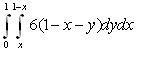# Integral and probability

• Cosmossos

## Homework Statement

The probability is given by:
p(x,y)=6(1-x-y)
x>=0
y>=0
x+y<=1

Show that p is normilized.
calculate <x>

## Homework Equations

I found that p is normilized using the integral:and It's equal to 1.
are the limits of the integral correct?

Why are you integrating $y$ from $x$ to $1-x$? Are you told that $y\geq x$? If not, you should be integrating from $0$ to $1-x$ instead.

I know that y>=x-1
and if I do it for 0 to 1 I don't get in the end that the probabilty =1

You were given $x+y \le 1$, so, moving the x over to the other side, you get $y \le 1-x$, right?

right... so that's why I chose the integral limits for dy to be from x to 1-x.
Is it wrong?

right... so that's why I chose the integral limits for dy to be from x to 1-x.
Is it wrong?

Again, why are you using $x$ as your lower limit? You are told that $y\geq0$, not $y\geq x$

I know that y>=x-1
and if I do it for 0 to 1 I don't get in the end that the probabilty =1

right... so that's why I chose the integral limits for dy to be from x to 1-x.
Is it wrong?
Your response to gabbagabbahey's question was confusing. In your previous post, you answered, it was because you knew $y \ge x-1$. The "x-1" suggested to me you somehow got that condition from the constraint $x+y \le 1$, and my point was that the constraint can only lead to $y \le 1-x$, the upper limit of the integral. There's no way you can get $y \ge x-1$ from it.

Even if you did have $y \ge x-1$, your answer didn't make sense. Why would the lower limit of the integral be x? Shouldn't it be x-1 if your inequality were true?